# Homotopy Type Theory and Higher Inductive Types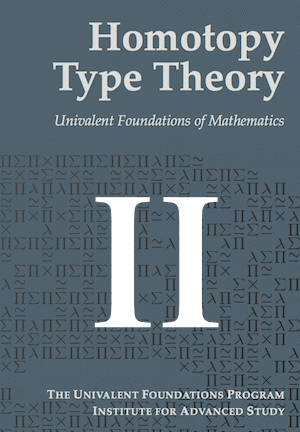In 2013, a consortium of the greatest mathematicians published a massive volume which reboots our conception of mathematics. Plus, in April 2014, Carnegie Mellon has received 7.5 million dollars from the Department of Defense to write the sequels of that book. In this article, we’ll dig into a new structure they’ve defined, which seems like a revolutionary and liberating breakthrough to me. This structure is that of higher inductive types, and it is the basis of homotopy type theory.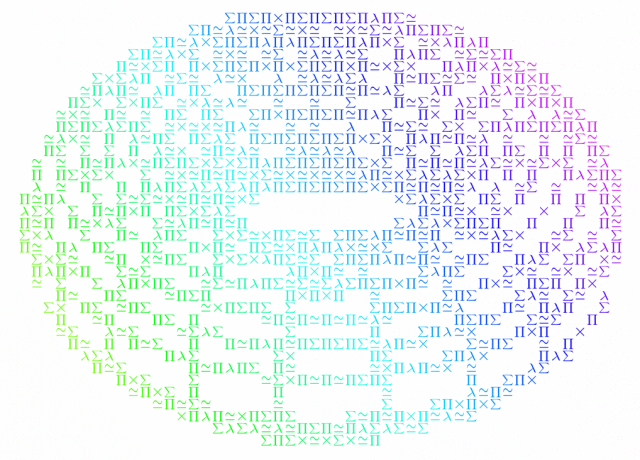$\require{color}$

The present article is the second of a series of 3 articles. The first part on type theory is not a prerequisite per se, but I would suggest you read it if you’re unfamiliar with issues of foundational mathematics. Also, beware that this article will be more technical than my usual articles. The third episode of this series is about the univalence axiom. If you don’t want to miss it, subscribe to Science4All with the box on the top right! Once again, I thank Andrew Cave for his guidance and corrections in my journey in the beautiful world of homotopy type theory.

## Previously, on Science4All…

But before getting to homotopy type theory, let’s have a quick recap on the essential elements of type theory. Type theory is the theory of types, which are structures made of terms. The type $\mathbb N$ of natural numbers is an example of such types, whose terms are natural numbers like $0$, $1$ and $1729$. We write $1729:\mathbb N$, to signify that $1729$ is a term (or inhabitant) of the type $\mathbb N$.

That sounds a lot like set theory…

There’s one big difference though. Types must be defined along with constructors. Every term of a type can only be defined by use of a constructor of the type. These constructors may also be regarded as the incoming functions of a type. This means that any function that outputs an element of a type must involve a constructor of that type. This perspective on types yields a formidably computable approach to mathematics.

So, type theory is all about constructions…

Yes. Well, we must also have a way to use the constructed terms of a type to define outgoing functions. This other side of the coin is known as the induction principle. Schematically, we have the following figure: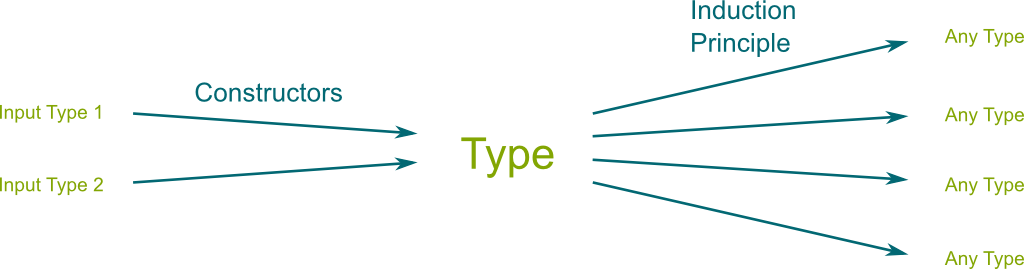I’ve found it useful to regard terms of types as the full sequence of constructions that led to them. Then, we have a direct way to assert equality: Two terms $x$ and $y$ are definitionally equal (or judgmentally) if they are the same sequence of constructions. We denote $x \equiv y$. Clearly, by definition, $x \equiv x$.

So, if I get it right, in type theory, two objects which are constructed differently are necessarily unequal, aren’t they?

They’d be definitionally unequal indeed. But, as Poincaré asserted it, “mathematics is the art of giving the same name to different things”. So, in many cases, we might want to prove the propositional equality of two definitionally unequal terms. More precisely, type theory defines the propositional equality of two terms $x$ and $y$ as a type $(x=y)$ whose terms are proofs of the equality. In other words, proving the propositional identity $x=y$ boils down to constructing an inhabitant $p$ of the type $(x=y)$, which we denote $p: (x=y)$, or, more simply, $p:x=y$.

How can we construct such proofs of propositional equalities?

First, we have a reflexivity proof $\mathsf{refl}_x : x = x$ derived from any definitional equality $x \equiv x$. Plus, we can use proofs to derive other proofs. In particular, using the induction principle of identity paths, we have the important action-on-path lemma: For any function $f$, and any two terms $x$ and $y$, we can construct a function $\mathsf{ap}_f : (x=y) \rightarrow (f(x) = f(y))$. This function $\mathsf{ap}_f$ constructs a proof of $\mathsf{ap}_f(p) : f(x) = f(y)$ from any proof $p:x=y$. Written informally, this says that the propositional identity $x=y$ implies the propositional identity $f(x)=f(y)$. More generally, an implication is now just a function!

## Integer Numbers

Now, a big portion of homotopy type theory builds upon the univalence axiom which I’ll only get to in the third part of this series. But there’s already a beautiful universe to explore without that univalence axiom. And it’s all based on higher inductive types.

What’s a higher inductive type?

In classical type theory, constructors could only construct terms of types. But, in an impulse of freeing us from that rigid law, homotopy type theorists have proposed to allow for the constructions of propositional identities as well. And this turned out to be extremely empowering!

Why?

Let me show you that with an example of construction of the type of integer numbers. A famous approach consists in defining $\mathbb Z$ as a quotient set of $\mathbb N \times \mathbb N$. I discussed it in my article on the set-theoretical construction of numbers, and its type-theoretical counterpart is quite interesting, as it already involves higher inductive types. Informally, this construction identifies pairs $(a,b)$ with the integer number “$a-b$”. Here’s an illustration of that construction.

Yet, I don’t know about you, but that does not look like a very natural construction to me. I mean, when you actually use integers, do you really picture them as equivalence classes of the Cartesian square of natural numbers? Surely, that works, but that’s not how informal mathematics works. Fortunately, one of the designated goals of homotopy type theory is to allow for more natural definitions of objects, hence making formal mathematics more like informal mathematics!

What I’m proposing here is not from the homotopy type theory book, which defines integers accordingly to the figure above. In fact, here, we’ll get a type which is not “the same” as the integer type $\mathbb Z$ of the book, as our type will not be a “set”. But I find our type so much more insightful to understand both integers and higher inductive types.
What would be a natural definition of integers?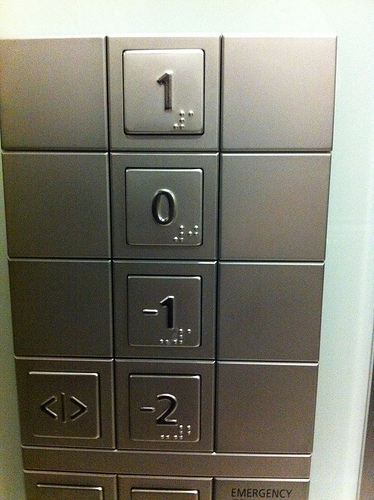I don’t know about you, but, back when I was in elementary school, I found the image of the (European) elevator very useful. In this metaphor, numbers are floors of an (infinite) elevator. And, because the elevator can move down, below the ground floor, we can get to negative numbers. More precisely, elevators have a one-floor-down move and a one-floor-up move. Interestingly, using these moves, we can get to any integer floor by starting at ground floor… Do you see the analogy with a type of integers?

I guess so… The moves correspond to constructors, don’t they?

Exactly! More precisely, the elevator-floor type $\mathsf{Floor}$ will have three constructors:

• The zero constructor $0:\mathsf{Floor}$.
• The up constructor $\color{OliveGreen} \uparrow: \mathsf{Floor} \rightarrow \mathsf{Floor}$, which gets us one floor up.
• The down constructor $\color{NavyBlue} \downarrow: \mathsf{Floor} \rightarrow \mathsf{Floor}$, which gets us one floor down.

So, intuitively, we’d have ${\color{OliveGreen} \uparrow}(0)$ being the “1” floor, while ${\color{NavyBlue} \downarrow} (0)$ would be the “-1” floor. But if our definition of constructors of $\mathsf{Floor}$ stopped here, there’d be one big trouble. If we go up then down, we wouldn’t end up at 0. In formal words, the term ${\color{NavyBlue} \downarrow}( {\color{OliveGreen} \uparrow} (0))$ here wouldn’t equal $0$.

Let me guess… We’re going to add a constructor of propositional identities!

Exactly! More precisely, let’s add the two identity constructors:

• For all $n : \mathsf{Floor}$, we have an identity constructor $\mathsf{id_{{\color{OliveGreen} \uparrow} {\color{NavyBlue} \downarrow}}}(n) : n = {\color{OliveGreen} \uparrow}({\color{NavyBlue} \downarrow}(n))$, which proves that going down then up is equivalent to staying still.
• For all $n : \mathsf{Floor}$, we have an identity constructor $\mathsf{id_{{\color{NavyBlue} \downarrow} {\color{OliveGreen} \uparrow}}}(n) : n = {\color{NavyBlue} \downarrow}({\color{OliveGreen} \uparrow}(n))$, which proves that going up then down is equivalent to staying still..If you're brought one floor down, but then get uplift one up... Well, guess what! You're back to square 1 (or, rather, floor 0).
If you’re familiar with homotopy type theory, you’ve probably noticed that this definition necessarily yields a complex homotopy of $\mathsf{Floor}$. In particular, $\mathsf{Floor}$ here is not a “set”. Obviously, a set-truncation enables to reduce $\mathsf{Floor}$ to a set, or we could have added two-dimensional proofs of $q=r$ for any two proofs $q,r:x=y$, but I also actually find it more natural to regard integers as the complex homotopy type $\mathsf{Floor}$. After all, when we reason with the elevator metaphor, there usually are different proofs that two sequences of up or down moves will lead to the same floor. But we’ll get to that in a bit… Finally, as pointed out by Vasili Galchin in private messages, this definition of integers is similar to presentations of groups.

To complete the definition of our type $\mathsf{Floor}$, we now need an induction principle to construct functions $f:\mathsf{Floor} \rightarrow A$ going out of $\mathsf{Floor}$. Like for $\mathbb N$, we first need the image $f(0):A$ of $0:\mathsf{Floor}$. Then, we need to know how to construct $f({\color{OliveGreen} \uparrow}(n)) : A$ from $f(n) : A$, which is done by a function $g_{\color{OliveGreen} \uparrow} : A \rightarrow A$. Similarly, to construct $f({\color{NavyBlue} \downarrow}(n))$ from $f(n)$, we need a function $g_{\color{NavyBlue} \downarrow} : A \rightarrow A$.

Wait a minute… If we stop here, we might have $f(0) \neq f({\color{NavyBlue} \downarrow}({\color{OliveGreen} \uparrow}(0)))$, right?

Excellent remark! We need to make sure that equal floors have equal images. This corresponds to determining proofs $\mathsf{id}^g_{{\color{OliveGreen} \uparrow} {\color{NavyBlue} \downarrow}}(x) : x = g_{\color{OliveGreen} \uparrow}(g_{\color{NavyBlue} \downarrow}(x))$ and $\mathsf{id}^g_{{\color{NavyBlue} \downarrow} {\color{OliveGreen} \uparrow}}(x) : x = g_{\color{NavyBlue} \downarrow} (g_{\color{OliveGreen} \uparrow} (x))$.

Are we done?

Yes! Given $f(0)$, $g_{\color{OliveGreen} \uparrow}$, $g_{\color{NavyBlue} \downarrow}$, $\mathsf{id}^g_{{\color{OliveGreen} \uparrow} {\color{NavyBlue} \downarrow}}$ and $\mathsf{id}^g_{{\color{NavyBlue} \downarrow} {\color{OliveGreen} \uparrow}}$, we now have a function $f: \mathsf{Floor} \rightarrow A$.

Formally, we’ve defined here the recursion principle $\mathsf{rec} : (A \times \sum \limits_{g_{\color{OliveGreen} \uparrow}:A \rightarrow A} \sum \limits_{g_{\color{NavyBlue} \downarrow}:A \rightarrow A} \prod \limits_{x:A} ((x=g_{\color{OliveGreen} \uparrow}(g_{\color{NavyBlue} \downarrow}(x))) \times (x=g_{\color{NavyBlue} \downarrow}(g_{\color{OliveGreen} \uparrow}(x)))) \rightarrow (\mathsf{Floor} \rightarrow A)$. The induction principle is much more lengthy to write, as it requires to deal with transports. But if you really want to know homotopy type theory, I invite you to do it as an exercise!

## Identity Proofs are Paths!

Now, intuitively, the rules we have introduced for our type $\mathsf{Floor}$ yield several different constructions of floor “$1$” in $\mathsf{Floor}$. This intuition is true. For instance, ${\color{OliveGreen} \uparrow}({\color{NavyBlue} \downarrow}({\color{NavyBlue} \downarrow}({\color{OliveGreen} \uparrow}({\color{OliveGreen} \uparrow}(0)))))$ equals ${\color{OliveGreen} \uparrow}(0)$. Let’s prove it! To simplify notations, from now on, I’ll drop parentheses when composing constructors ${\color{OliveGreen} \uparrow}$ and ${\color{NavyBlue} \downarrow}$, and the 0 which is always the first input term of any sequence of constructors. So, with these notations, what we want to prove, is ${\color{OliveGreen} \uparrow} {\color{NavyBlue} \downarrow} {\color{NavyBlue} \downarrow} {\color{OliveGreen} \uparrow} {\color{OliveGreen} \uparrow} = {\color{OliveGreen} \uparrow}$.

Well, basically, whenever we have ${\color{OliveGreen} \uparrow} {\color{NavyBlue} \downarrow}$ or ${\color{NavyBlue} \downarrow} {\color{OliveGreen} \uparrow}$, we can remove these, right?

That’s the idea! Let’s start by “removing” the first ${\color{OliveGreen} \uparrow} {\color{NavyBlue} \downarrow}$ of the term ${\color{OliveGreen} \uparrow} {\color{NavyBlue} \downarrow} {\color{NavyBlue} \downarrow} {\color{OliveGreen} \uparrow} {\color{OliveGreen} \uparrow}$. I mean, let’s verify that ${\color{NavyBlue} \downarrow} {\color{OliveGreen} \uparrow} {\color{OliveGreen} \uparrow} = {\color{OliveGreen} \uparrow} {\color{NavyBlue} \downarrow} ({\color{NavyBlue} \downarrow} {\color{OliveGreen} \uparrow} {\color{OliveGreen} \uparrow} )$. Well, that’s given by the proof constructor $\mathsf{id_{{\color{OliveGreen} \uparrow} {\color{NavyBlue} \downarrow}}} ({\color{NavyBlue} \downarrow} {\color{OliveGreen} \uparrow} {\color{OliveGreen} \uparrow})$! Next, we need remove the first ${\color{NavyBlue} \downarrow} {\color{OliveGreen} \uparrow}$ of the expression ${\color{NavyBlue} \downarrow} {\color{OliveGreen} \uparrow} {\color{OliveGreen} \uparrow}$. And that’s given by the proof $\mathsf{id_{{\color{NavyBlue} \downarrow} {\color{OliveGreen} \uparrow}}}({\color{OliveGreen} \uparrow}) : {\color{OliveGreen} \uparrow} = {\color{NavyBlue} \downarrow} {\color{OliveGreen} \uparrow} {\color{OliveGreen} \uparrow}$. So, to recapitulate, we have proofs that ${\color{NavyBlue} \downarrow} {\color{OliveGreen} \uparrow} {\color{OliveGreen} \uparrow} = {\color{OliveGreen} \uparrow} {\color{NavyBlue} \downarrow} {\color{NavyBlue} \downarrow} {\color{OliveGreen} \uparrow} {\color{OliveGreen} \uparrow}$ and ${\color{OliveGreen} \uparrow} = {\color{NavyBlue} \downarrow} {\color{OliveGreen} \uparrow} {\color{OliveGreen} \uparrow}$. But can we use these to construct a proof of ${\color{OliveGreen} \uparrow} {\color{NavyBlue} \downarrow} {\color{NavyBlue} \downarrow} {\color{OliveGreen} \uparrow} {\color{OliveGreen} \uparrow} = {\color{OliveGreen} \uparrow}$?

Hummm… Isn’t it obvious? Although, I’m not sure how to prove it formally…

Here we get to the beautiful central idea of homotopy type theory! Proofs $q : x = y$ may be interpreted as paths from $x$ to $y$. Given this, terms of types can now also called points in spaces. And, using the path induction principle, it can be proved that paths can be composed. Like in homotopy theory! This means that if $q: x = y$ and $r:y=z$, then we can construct a composed path $q \cdot r : x=z$. Similarly, if $q:x=y$, we can invert $q$, hence constructing $q^{-1} : y=x$.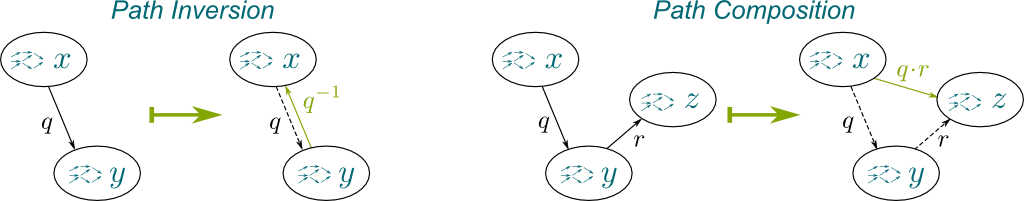Let’s apply that to prove ${\color{OliveGreen} \uparrow} {\color{NavyBlue} \downarrow} {\color{NavyBlue} \downarrow} {\color{OliveGreen} \uparrow} {\color{OliveGreen} \uparrow} = {\color{OliveGreen} \uparrow}$. Recall that $\mathsf{id_{{\color{OliveGreen} \uparrow} {\color{NavyBlue} \downarrow}}}({\color{NavyBlue} \downarrow} {\color{OliveGreen} \uparrow} {\color{OliveGreen} \uparrow}) : {\color{NavyBlue} \downarrow} {\color{OliveGreen} \uparrow} {\color{OliveGreen} \uparrow} = {\color{OliveGreen} \uparrow} {\color{NavyBlue} \downarrow} {\color{NavyBlue} \downarrow} {\color{OliveGreen} \uparrow} {\color{OliveGreen} \uparrow}$. We can invert this path, yielding $\mathsf{id_{{\color{OliveGreen} \uparrow} {\color{NavyBlue} \downarrow}}}({\color{NavyBlue} \downarrow} {\color{OliveGreen} \uparrow} {\color{OliveGreen} \uparrow})^{-1} : {\color{OliveGreen} \uparrow} {\color{NavyBlue} \downarrow} {\color{NavyBlue} \downarrow} {\color{OliveGreen} \uparrow} {\color{OliveGreen} \uparrow} = {\color{NavyBlue} \downarrow} {\color{OliveGreen} \uparrow} {\color{OliveGreen} \uparrow}$. Similarly, $\mathsf{id_{{\color{NavyBlue} \downarrow} {\color{OliveGreen} \uparrow}}}( {\color{OliveGreen} \uparrow})^{-1} : {\color{NavyBlue} \downarrow} {\color{OliveGreen} \uparrow} {\color{OliveGreen} \uparrow} = {\color{OliveGreen} \uparrow}$. Finally, using path composition, we have the proof $\mathsf{id_{{\color{OliveGreen} \uparrow} {\color{NavyBlue} \downarrow}}}({\color{NavyBlue} \downarrow} {\color{OliveGreen} \uparrow} {\color{OliveGreen} \uparrow})^{-1} \cdot \mathsf{id_{{\color{NavyBlue} \downarrow} {\color{OliveGreen} \uparrow} }}( {\color{OliveGreen} \uparrow})^{-1} : {\color{OliveGreen} \uparrow} {\color{NavyBlue} \downarrow} {\color{NavyBlue} \downarrow} {\color{OliveGreen} \uparrow} {\color{OliveGreen} \uparrow} = {\color{OliveGreen} \uparrow}$. We’ve done it!

Cool!

Now, what’s amazing as we view proofs as paths is that we can see the “different” terms of the type $\mathsf{Floor}$ as the different connected components. Let’s draw it: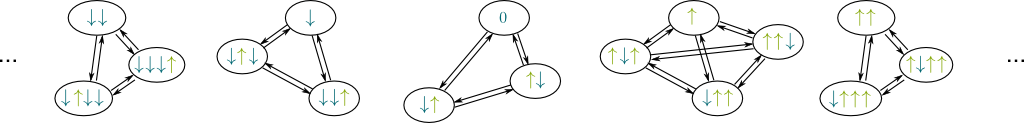And that’s where things become very interesting from a homotopical viewpoint!

## Homotopy Groups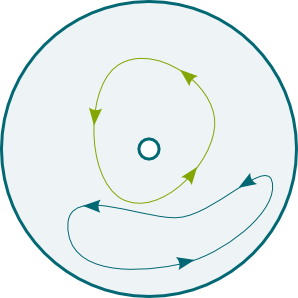The pinnacle of homotopy theory is Perelman’s recent proof of the Poincaré conjecture, which states that, for $n \geq 2$, all $n$-spheres are topologically characterized by the triviality of their homotopy group.

What? What the hell does that mean?

You may read my article on homotopy to learn more, but, for our purpose, what it means is that homotopy is a cornerstone of modern mathematics. In particular, homotopy groups, also known as fundamental group, are, well, fundamental.

What are these?

Roughly, a homotopy group is a set of different loops. Interpreted in terms of homotopy type theory, this homotopy group is the set of different proofs of an identity between two identical constructions.

You might have noticed that I’m here talking about the set of different proofs – not the type. Sets in homotopy type theory are types with no higher homotopy, in the sense that there always is only one proof of an identity. By opposition the pointed loop type (or space) $\Omega(\mathsf{Floor}, {\color{OliveGreen} \uparrow})$ is defined as the type ${\color{OliveGreen} \uparrow} = {\color{OliveGreen} \uparrow}$, and there may be different (so-called 2-dimensional) paths between two paths of the loop type.
Hummm… Can you give an example?

Sure! Our definition of the type $\mathsf{Floor}$ will provide a great one. Let’s give two different proofs that ${\color{OliveGreen} \uparrow} = {\color{OliveGreen} \uparrow} {\color{NavyBlue} \downarrow} {\color{OliveGreen} \uparrow}$. As you’ll see, this will lead us to two different paths ${\color{OliveGreen} \uparrow} = {\color{OliveGreen} \uparrow}$.

Well, to prove ${\color{OliveGreen} \uparrow} = {\color{OliveGreen} \uparrow} {\color{NavyBlue} \downarrow} {\color{OliveGreen} \uparrow}$, we can remove the two first arrows…

Yes. This corresponds to the proof $\mathsf{id}_{{\color{OliveGreen} \uparrow} {\color{NavyBlue} \downarrow}} ({\color{OliveGreen} \uparrow}) : {\color{OliveGreen} \uparrow} = {\color{OliveGreen} \uparrow} {\color{NavyBlue} \downarrow} {\color{OliveGreen} \uparrow}$. But we can work out the equality in a different way. Do you see it?

Well, we can remove the two last arrows instead…

Exactly. To do so, let’s forget about the first arrow for a second, and prove ${\color{NavyBlue} \downarrow} {\color{OliveGreen} \uparrow} = 0$. That’s given by $\mathsf{id}_{{\color{NavyBlue} \downarrow} {\color{OliveGreen} \uparrow}}(0) : 0 = {\color{NavyBlue} \downarrow} {\color{OliveGreen} \uparrow}$. We can now derive the former identity by applying the up constructor to both sides. By the action-on-path lemma, we then have a proof $\mathsf{ap}_{\color{OliveGreen} \uparrow} (\mathsf{id}_{{\color{NavyBlue} \downarrow} {\color{OliveGreen} \uparrow}}(0) ) : {\color{OliveGreen} \uparrow} = {\color{OliveGreen} \uparrow} {\color{NavyBlue} \downarrow} {\color{OliveGreen} \uparrow}$. And, while it’s actually not formally straightforward, intuitively, the proof $\mathsf{ap}_{\color{OliveGreen} \uparrow} (\mathsf{id}_{{\color{NavyBlue} \downarrow} {\color{OliveGreen} \uparrow}}(0) )$ is different from $\mathsf{id}_{{\color{OliveGreen} \uparrow} {\color{NavyBlue} \downarrow}} ({\color{OliveGreen} \uparrow})$, as it comes from a completely different proof construction.

In fact, it’s so not straightforward that it’s actually wrong in some models of type theory where all paths are equal. To prove that these proofs are different paths require some additional axiom, like the univalence axiom.
You said it would yield two different proofs of ${\color{OliveGreen} \uparrow} = {\color{OliveGreen} \uparrow}$… How so?

Our two different proofs can now be regarded to different paths ${\color{OliveGreen} \uparrow} = {\color{OliveGreen} \uparrow} {\color{NavyBlue} \downarrow} {\color{OliveGreen} \uparrow}$. This means that we can go from ${\color{OliveGreen} \uparrow}$ to ${\color{OliveGreen} \uparrow} {\color{NavyBlue} \downarrow} {\color{OliveGreen} \uparrow}$ with one path, and come back to ${\color{OliveGreen} \uparrow}$ with the other path. Formally, this loop is the path composition $\mathsf{id}_{{\color{OliveGreen} \uparrow} {\color{NavyBlue} \downarrow}} ({\color{OliveGreen} \uparrow}) \cdot \mathsf{ap}^{-1}_{\color{OliveGreen} \uparrow} (\mathsf{id}_{{\color{NavyBlue} \downarrow} {\color{OliveGreen} \uparrow}}(0) )$, and it represents a non-trivial proof of ${\color{OliveGreen} \uparrow} = {\color{OliveGreen} \uparrow}$.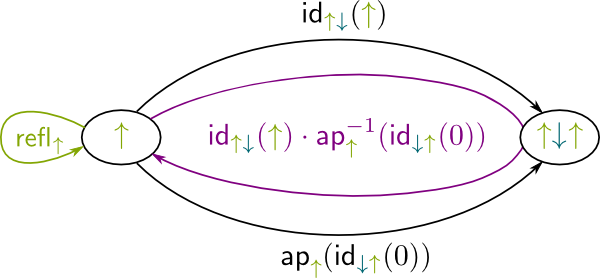Just like in homotopy theory, the homotopy group $\pi_1(\mathsf{Floor}, {\color{OliveGreen} \uparrow})$ centered on ${\color{OliveGreen} \uparrow}$ is the set of different paths (or proofs) ${\color{OliveGreen} \uparrow} = {\color{OliveGreen} \uparrow}$. But, crucially, it does not really depend on the point it is centered on, but rather on the connected component which contains that point. This is because $\pi_1(\mathsf{Floor}, {\color{OliveGreen} \uparrow})$ is isomorphic to $\pi_1(\mathsf{Floor}, {\color{OliveGreen} \uparrow} {\color{NavyBlue} \downarrow} {\color{OliveGreen} \uparrow})$, in the sense that there is a natural one-to-one map between these two homotopy groups.

More precisely, if $p:x=y$, then we can switch viewpoint from $x$ to $y$ through the isomorphism $\pi_1(x) \simeq \pi_1(y)$ defined by $loop \mapsto p^{-1} \cdot loop \cdot p$.

Now, the homotopy group $\pi_1(\mathsf{Floor}, {\color{OliveGreen} \uparrow})$ here is actually quite complicated, and I’m not going to venture describing it here. Instead, let’s focus on basic homotopical spaces!

## Circles and Spheres

What would be the simplest higher inductive type with a non trivial homotopy? Well, let’s just have a single constructor of a single term, and a single constructor of a single non-trivial path. We’ll call that type $\mathbb S^1$. Here are its constructors:

• The constructor of the base term defined by $\mathsf{base} : \mathbb S^1$.
• The constructor of a (non-trivial) loop defined by $\mathsf{loop} : \mathsf{base} = \mathsf{base}$.

Let’s draw the homotopy of $\mathbb S^1$.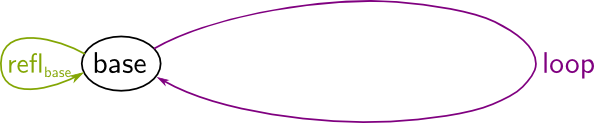Note that in this homotopical perspective, the trivial proof $\mathsf{refl_{base}}$ stands for staying still at $\mathsf{base}$. Now, In the figure above, it’s useful to imagine that there’s a whole inside the $\mathsf{loop}$, so that the $\mathsf{loop}$ cannot be continuously deformed into the $\mathsf{refl_{base}}$. Doesn’t this structure remind you of something?

A circle?

Exactly! As weird as it sounds, the constructors we have given above define the (homotopical) circle $\mathbb S^1$. I bet you’ve never thought of circles that way!

Waw! That is… weird!

I know! It’s also a much cleaner definition of the circle than any other I’ve ever read before. This is especially true when you ponder the fact that there is not always an equivalent of the usual real numbers in constructive mathematics! Similarly, the mere definition of topology in constructive mathematics is very tricky. Given that, it’s not obvious how homotopy theory could be developed constructively, and how proofs in homotopy theory could be computationally checked. Amazingly, higher inductive types and homotopy type theory give us a clean, simple and elegant model for homotopy theory!

So what would be the homotopy group of the circle?

Intuitively, any path in a circle consists in looping with regards to the $\mathsf{base}$ clockwisely or anti-clockwisely. Plus, we can remark that looping once clockwisely and then once anti-clockwisely is equivalent to staying at the $\mathsf{base}$ all along. In other words, $\mathsf{loop} \cdot \mathsf{loop}^{-1} = \mathsf{refl}_0$. So, intuitively, any path has the form $\mathsf{loop}^n$ with $n \in \mathbb Z$.

Formally, identities $\mathsf{loop} \cdot \mathsf{loop}^{-1} = \mathsf{refl}_0$ and $\mathsf{loop}^{-1} \cdot \mathsf{loop} = \mathsf{refl}_0$ are derived from the path induction principle.
So, the fundamental group $\pi_1(\mathbb S^1)$ would be isomorphic to $\mathbb Z$, right?

It seems obvious. Yet, weirdly enough, we cannot prove it without additional axiom! So, the proof of $\pi_1(\mathbb S^1) = \mathbb Z$ will have to wait for the third part of this series, where we’ll present the univalence axiom!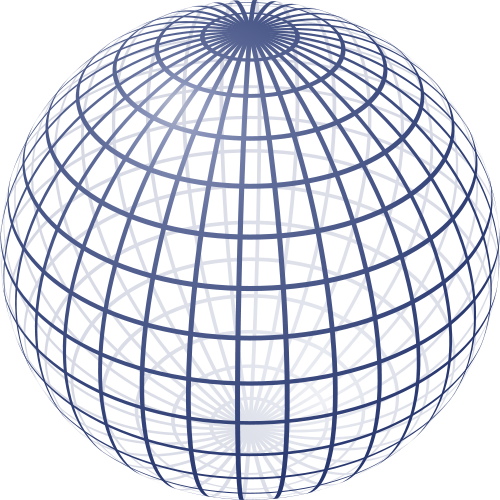To conclude this section, let me blow your mind with the most amazing definition I have found in this book.

What’s that definition?

It’s the definition of the sphere $\mathbb S^2$. What’s a sphere? Well, in homotopy type theory, it’s the type defined by the two following constructors:

• A base point constructor $\mathsf{base} : \mathbb S^2$.
• A (non-trivial) 2-dimensional path constructor $\mathsf{surface} : \mathsf{refl_{base}} = \mathsf{refl_{base}}$.

In other words, the sphere is defined as a point with a non-trivial loop of loop from staying at $\mathsf{base}$ to staying at $\mathsf{base}$.

Waw! That’s really messing with my head!

It should! But reflecting on this spectacularly clean but awfully complex structure is the gateway to grasping the key ideas of homotopy type theory. That’s why I’m ending this article here!

## Conclusion

The introduction of higher inductive types starts with a simple idea: Let’s allow the construction of identity proofs! As mathematics has developed into a constructive art, and while mathematicians are asking for more rights to create, such a liberating authorization may turn out to be a cornerstone for an upcoming blow-up of new mathematical ideas. In this article, it’s enabled me to write down a very intuitive construction of integers, which much better fits the intuition we (or at least I) first had about them! But, more importantly, for the top mathematicians at the forefront of the (computable) mathematical frontier, higher inductive type has been an amazing basis to cleanly and elegantly approach homotopy theory from a much more direct way.

However, in this article, there is one missing weapon that our revolution will need to be successful (as far as we know). This weapon is the elegant univalence axiom, introduced by Fields medalist Vladimir Voevodsky. In the third and last episode of this series, we’ll see how successful Voevodsky’s weapon may be (or already is!) at reshaping the landscape of mathematics.